Get instant live expert help with Excel or Google Sheets“My Excelchat expert helped me in less than 20 minutes, saving me what would have been 5 hours of work!”

#### Post your problem and you'll get expert help in seconds

Your message must be at least 40 characters
Our professional experts are available now. Your privacy is guaranteed.

# Count times in a specific rangeRead time: 25 minutes

While working with Excel, we are able to determine the number of values that fall within a specified range by using the COUNTIFS function. The COUNTIFS function counts the number of cells that meet one or more criteria.  This step by step tutorial will assist all levels of Excel users in counting times in a specific range with COUNTIFS.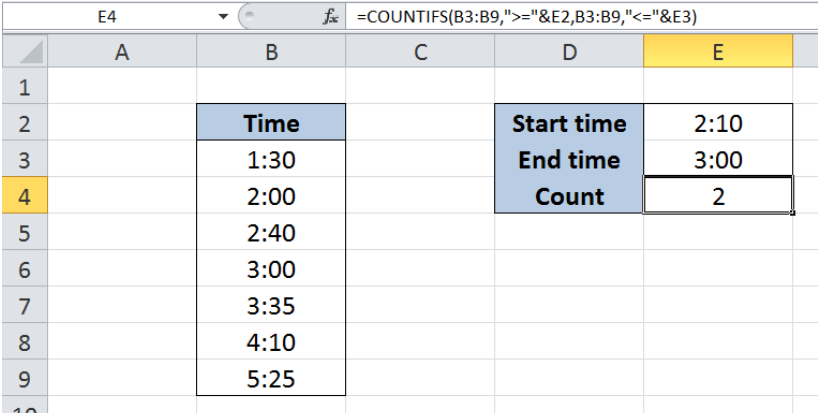Figure 1. Final result: Count times in a specific range

Final formula using cell reference in criteria: `=COUNTIFS(B3:B9,">="&E2,B3:B9,"<="&E3)`

Final formula with hard-coded values in criteria: `=COUNTIFS(B3:B9,">=2:10",B3:B9,"<=3:00")`

## Syntax of COUNTIFS Function

`=COUNTIFS(criteria_range1, criteria1, [criteria_range2, criteria2]…)  `

Parameters:

• Criteria_range1: the data range that will be evaluated using the criteria1
• Criteria1:  the criteria or condition that determines which cells will be counted
• Criteria_range2 and criteria2 are optional; only applied when there are more than one criteria as specified

## Setting up Our Data

Our table consists of one column containing Time (column B).  Cells E2 and E3 contain our criteria, with start time of “2:10” and end time of “3:00”.  We want to count the number of times within the given specific range. The resulting count will be in cell E4.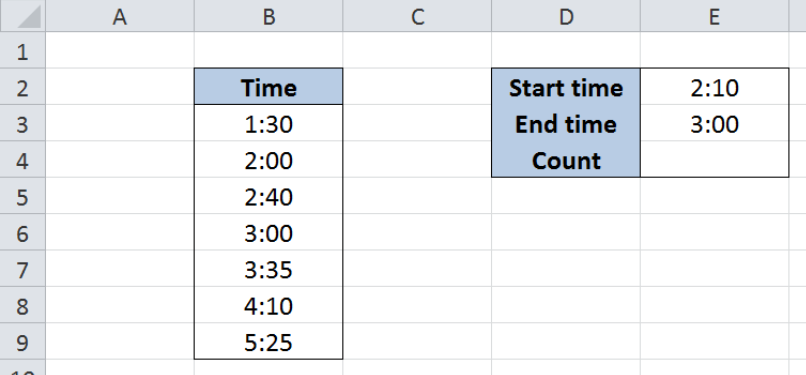Figure 2. Sample data to count times in a specific range

## Count times in a specific range

We want to determine the number of times in the list which fall between 2:10 and 3:00.

To count the number of times within the specific range, we will follow these steps:

Step 1.  Select cell E4

Step 2.  Enter the formula: `=``COUNTIFS(B3:B9,">="&E2,B3:B9,"<="&E3)`

Step 3: Press ENTER

The range for our data set is B3:B9.  Our formula has two criteria.  It counts the cells in column B  with values between 2:10 and 3:00.  The symbol “>=” means “greater than or equal to” while “<=” means “less than or equal to”.  The ampersand “&” links the symbols with our start time and end time.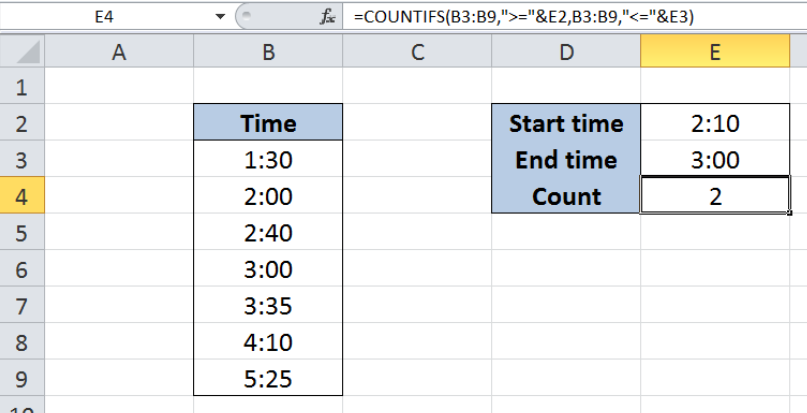Figure 3. Using the COUNTIFS function to count times between 2:10 and 3:00

Our formula returns the value 2, which corresponds to the two cells with values between 2:10 and 3:00 as highlighted below.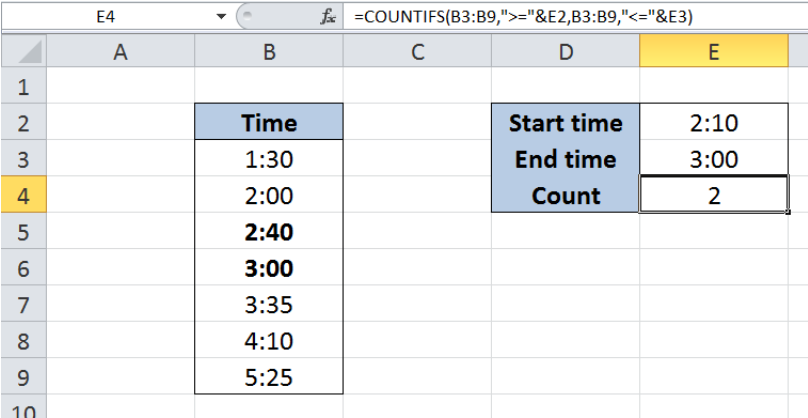Figure 4. Highlighting the times between 2:10 and 3:00

## Note:

Aside from using cell references, we can also input the criteria directly into our formula by hardcoding the values for start time and end time.  Let us follow below steps:

Step 1.  Select cell E4

Step 2.  Enter the formula: `=``COUNTIFS(B3:B9,">=2:10",B3:B9,"<=3:00")`

Step 3: Press ENTER

The result in cell E4 is 2, which is the same as in our previous example.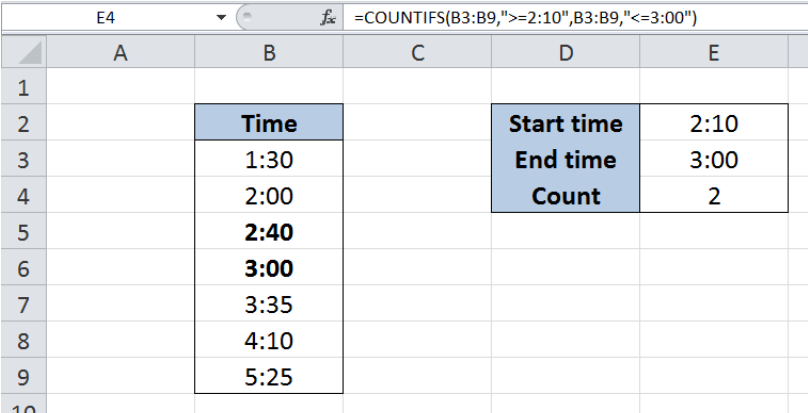Figure 5. Hardcoding the criteria for times into the COUNTIFS formula

Most of the time, the problem you will need to solve will be more complex than a simple application of a formula or function. If you want to save hours of research and frustration, try our live Excelchat service! Our Excel Experts are available 24/7 to answer any Excel question you may have. We guarantee a connection within 30 seconds and a customized solution within 20 minutes.

### Did this post not answer your question? Get a solution from connecting with the expert.Another blog reader asked this question today on Excelchat:## Subscribe to Excelchat.coAnother blog reader asked this question today on Excelchat: